ASI寻址诊断多用表

4½位高性能万用表AM系列
4⁶∕₇ 位高性能万用表PM系列

1500V光伏测试仪

SMARTCOLLECT

OEM&ODM产品定制Top

>
>

# 功率分析仪知识科普：功率的定义（Power）p(t)=u(t)*i(t)

S=Utrms*Itrms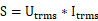功率波形的平均值。公式是：

P=1Tt=0Tut*itdt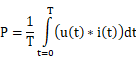P的正负号取决于功率的流向。有功功率仅由频率相同的电压和电流成

Q=S2-P2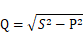1. 由相同频率的电压和电流成分之间的相位差引起“相移无功功率”（也称“位移无功功率”），符号Qshift，计算公式如下：

Qshift=i=1nUi*Ii*sinφ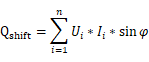1. 不同频率的电压和电流成分之间产生的功率称为“畸变无功功率”，用D或者Qdist表示。

Q=Qshift2+Qdist2=Qshift2+D2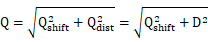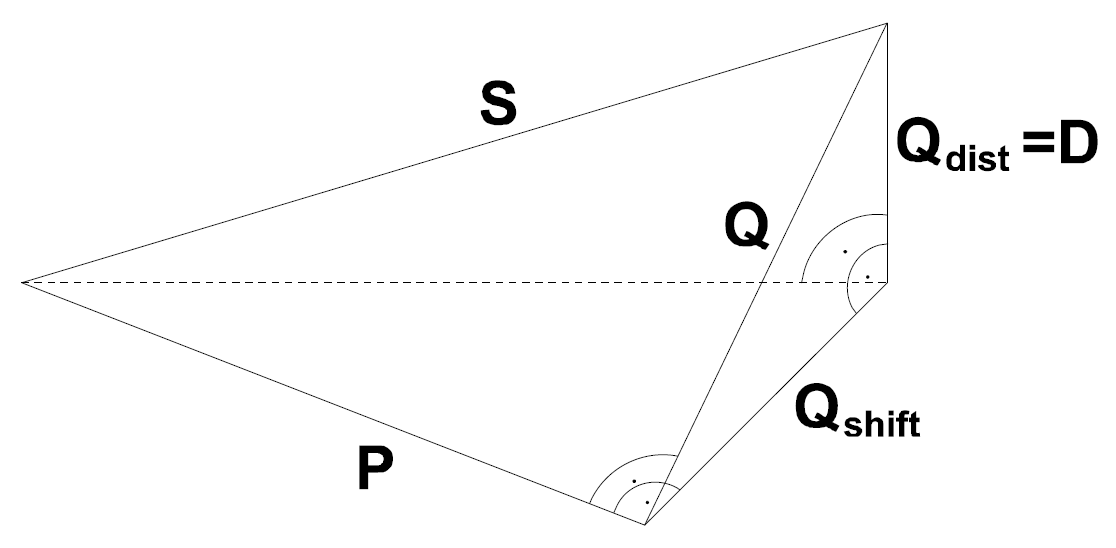1：不同功率之间的图形关系

D=S2-P2-Qshift2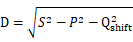规则一、相同频率的电压和电流仅能产生有功功率和相移无功功率。

pt=i*sinωt+φi*u*sinωt+φu=i*u*(12cosφi-φu-12cos2ωt+φi+φu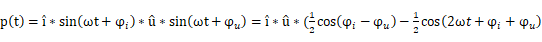)

i*u*12cosφi-φu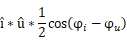i*u*12cos2ωt+φi+φu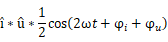pt=i*u*12cosφi-φu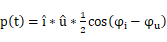=i2*u2*cosφi-φu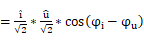= I*U*cos(φ)

pt=i*sinω1t+φi*u*sinω2t+φu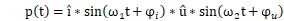=i*u*(12cos(ω1-ω2)*t+φi-φu-12cos(ω1+ω2)*t+φi+φu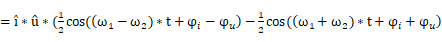)

T=1gcd⁡(f1,f2)1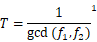1: gcd是最大公约数。此函数仅对整数有效。如果不是，则分数必需乘以一个公因数。

f1=50Hz, f2=50.2Hz, T=10/gcd(500,502)=5s